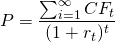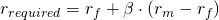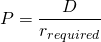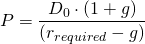# Dividend discount model

Equity valuation has its roots in fixed rate bond valuation. Just as with bonds, the value of equity is determined by discounting future cash flows using a discount rateThe difference between equity and bond valuation lies in the uncertainty about future cash flows. Whereas bond cash flows can be determined precisely with a high degree of certainty, this is not the case for equities. This is because shareholders are the residual owners of a company. They only obtain money after all other debtholders are paid; they are entitled to the profit after tax. Additionally, future cash flows are largely dependent on the future economic state and can thus vary a lot from year to year. Moreover, profit growth is also dependent on the company’s age and sector it is active in. For example, young companies active in technology obviously can grow more than mature companies active in retail.

## The required return

Discount factors used in equity valuation differ from those used in bond valuation. Equity will be discounted using a larger discount rate. This is because investors want to be compensated for the additional risk they are taking when holding equities. The discount factor can be determined using the Capital Asset Pricing Model (CAPM). As explained before, the required rate of return can be calculated using on the risk free rate, rf, the market return, rm, and the share’s systematic risk with respect to the market, ß.## Basic dividend discount model

The dividend discount model assumes that all future cash flows to the firm will be distributed to the shareholders in the form of dividends. The previous formula which discount cash flows till infinity can also be written as a perpetual. This leads to the following equivalent, but easier, formula. The value of a share equals the next dividend (here assumed being constant for eternity) divided by the required rate of return.## Model with growth

In reality, it is often the case that companies steadily increase their dividend over time.To account for this, the basic dividend discount model can be extended further. In the numerator, the last known dividend is multiplied by (1+g) which captures the growth in dividends. The denominator now contains, r-g, also a result of dividend growth (with r > g).## Summary

There’s more uncertainty in the valuation of equity than in those of bonds. The dividend discount model assumes cash flows being paid out as dividends and required a discount return which can be calculated based on capm. Also growth in dividends can have a significant positive effect.

### Dividend discount model

Need to have more insights? Download our free excel file: dividend discount model.